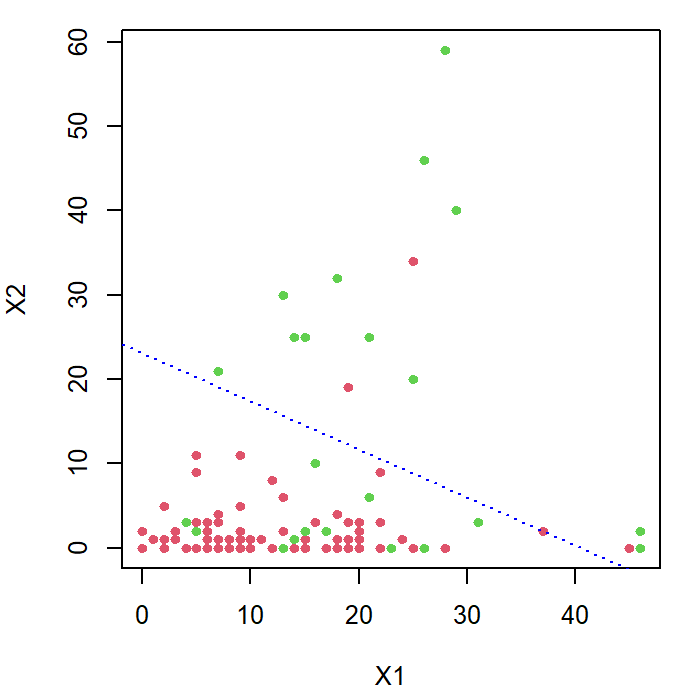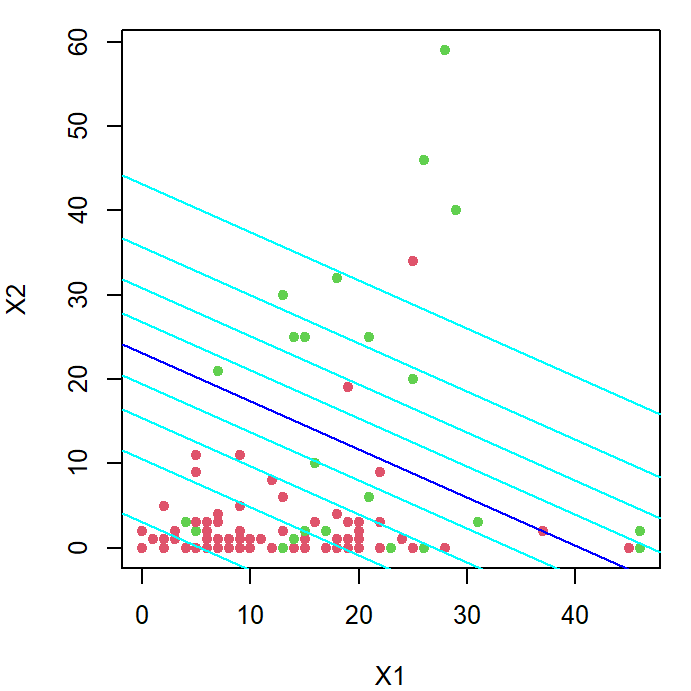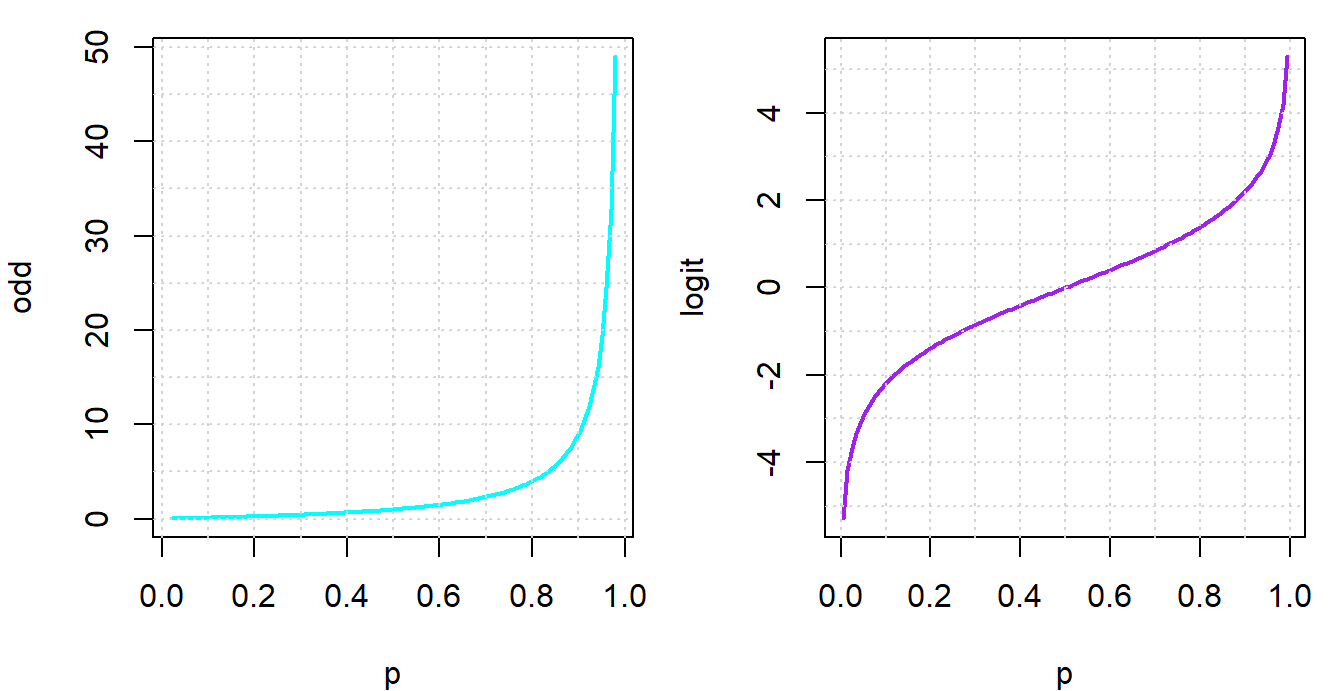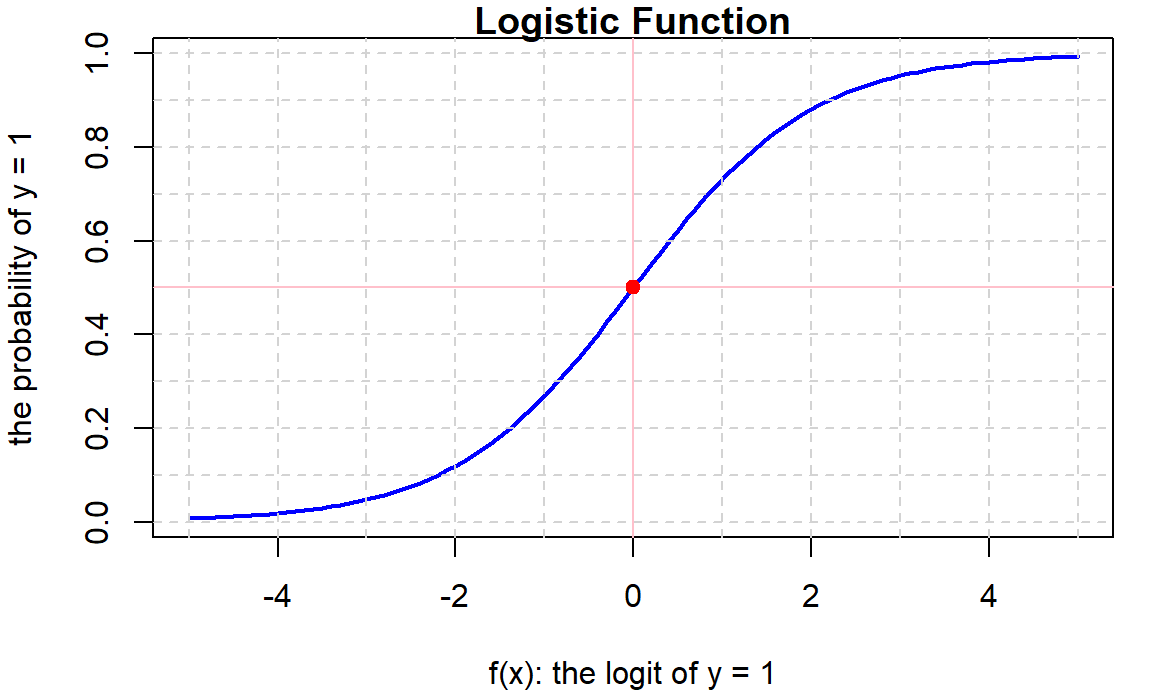pacman::p_load(ggplot2, dplyr)

🌻 Notes on Categorical Models :

• Models that predict categorical outcomes are referred as classifier
• Classifiers predict the probabilities of each categories
• Logistic Regression is a simple classifying method that only predicts binary outcomes
• Binary outcomes used to be encoded as ‘0’ and ‘1’
• Logistic regression models predict the Prob[y = 1]

### 【A】A Simple Starter Example

• predict an uncertain binary outcome y encoded as 0 & 1
• with two predictor variables x1 & x2
D = read.csv("data/quality.csv")  # Read in dataset
D = D[,c(14, 4, 5)]
names(D) = c("y", "x1", "x2")

Check the proportion of 1 in y. It implies the probability of y==1, if we randomly pick one data point from the dataset.

table(D$y)  0 1 98 33  🌻 The Method : Generalize Liner Model • glm(y~x1+x2, data, family=binomial) • family=bonomial specifies y is binary and the model predicts P[y=1] glm1 = glm(y~x1+x2, D, family=binomial) summary(glm1)  Call: glm(formula = y ~ x1 + x2, family = binomial, data = D) Deviance Residuals: Min 1Q Median 3Q Max -2.377 -0.627 -0.510 -0.154 2.119 Coefficients: Estimate Std. Error z value Pr(>|z|) (Intercept) -2.5402 0.4500 -5.64 0.000000017 *** x1 0.0627 0.0240 2.62 0.00892 ** x2 0.1099 0.0326 3.37 0.00076 *** --- Signif. codes: 0 '***' 0.001 '**' 0.01 '*' 0.05 '.' 0.1 ' ' 1 (Dispersion parameter for binomial family taken to be 1) Null deviance: 147.88 on 130 degrees of freedom Residual deviance: 116.45 on 128 degrees of freedom AIC: 122.4 Number of Fisher Scoring iterations: 5 coef extracts the coefficients from the model b = coef(glm1); b # extract the regression coef (Intercept) x1 x2 -2.540212 0.062735 0.109896  • The linear combination $$b_0 + b_1 x_1 + b_2 x_2$$ predicts the logit of y==1, it take a series of transformation to acquire the corresponding probability. • $$logit = f(x) = b_0 + b_1 x_1 + b_2 x_2$$ • $$odd = Exp(logit)$$ • $$Pr[y = 1] = prob = \frac{odd}{1+odd}$$ Given x1=3, x2=4, what are the predicted logit, odd and probability? logit = sum(b * c(1, 3, 4)) odd = exp(logit) prob = odd/(1+odd) c(logit=logit, odd=odd, prob=prob)  logit odd prob -1.91242 0.14772 0.12871  🗿 : What if x1=2, x2=3? logit = sum(b * c(1, 2, 3)) odd = exp(logit) prob = odd/(1+odd) c(logit=logit, odd=odd, prob=prob)  logit odd prob -2.08505 0.12430 0.11056  ### 【B】 Plot the Regrssion Line The method produce a linear combination of x’s that demarcates y=0 and y=1 in the space of x’s. We can plot the line of logit = 0 or odd = 1, prob = 0.5 on the plane of $$X$$ par(cex=0.8, mar=c(4,4,1,1)) plot(D$x1, D$x2, col=2+D$y, pch=20, cex=1.2, xlab="X1", ylab="X2")
abline(-b/b, -b/b, col="blue", lty=3)Furthermore, we can translate probability, logit and coefficients to intercept & slope …

$f(x) = b_0 + b_1 x_1 + b_2 x_2 \; \Rightarrow \; x_2 = \frac{f - b_0}{b_2} - \frac{b_1}{b_2}x_1$

p = seq(0.1,0.9,0.1)
logit = log(p/(1-p))
data.frame(prob = p, logit)
  prob    logit
1  0.1 -2.19722
2  0.2 -1.38629
3  0.3 -0.84730
4  0.4 -0.40547
5  0.5  0.00000
6  0.6  0.40547
7  0.7  0.84730
8  0.8  1.38629
9  0.9  2.19722

then mark the contours of proabilities into the scatter plot

par(cex=0.8, mar=c(4,4,1,1))
plot(D$x1, D$x2, col=2+D\$y,pch=20, cex=1.3, xlab='X1', ylab='X2')
for(f in logit) {
abline((f-b)/b, -b/b, col=ifelse(f==0,'blue','cyan')) }🗿 : What do the blue/cyan lines means?

🗿 : Given any point in the figure above, can you predict the probability (approximately)?

### 【C】 Odd, Logit and Logistic Functions

##### The trinity: probability, odd & logit
• Odd = $$p/(1-p)$$

• Logit = $$log(odd)$$ = $$log(\frac{p}{1=p})$$

• $$o = p/(1-p)$$ ; $$p = o/(1+o)$$ ; $$logit = log(o)$$

par(cex=0.8, mfcol=c(1,2))
curve(x/(1-x), 0.02, 0.98, col='cyan',lwd=2,
ylab='odd', xlab='p')
abline(v=seq(0,1,0.1), h=seq(0,50,5), col='lightgray', lty=3)
curve(log(x/(1-x)), 0.005, 0.995, lwd=2, col='purple',
ylab="logit",xlab='p')
abline(v=seq(0,1,0.1), h=seq(-5,5,1), col='lightgray', lty=3)##### Logistic Function & Logistic Regression
• Linear Model: $$y = f(x) = b_0 + b_1x_1 + b_2x_2 + ...$$

• General Linear Model(GLM): $$y = Link(f(x))$$

• Logistic Regression: $$logit(y) = log(\frac{p}{1-p}) = f(x) \text{ where } p = prob[y=1]$$

• Logistic Function: $$Logistic(F_x) = \frac{1}{1+Exp(-F_x)} = \frac{Exp(F_x)}{1+Exp(F_x)}$$

par(cex=0.8, mfrow=c(1,1))
curve(1/(1+exp(-x)), -5, 5, col='blue', lwd=2,main="Logistic Function",
xlab="f(x): the logit of y = 1", ylab="the probability of y = 1")
abline(v=-5:5, h=seq(0,1,0.1), col='lightgray', lty=2)
abline(v=0,h=0.5,col='pink')
points(0,0.5,pch=20,cex=1.5,col='red')🗿 : What are the definiion of logit & logistic function? What is the relationship between them?